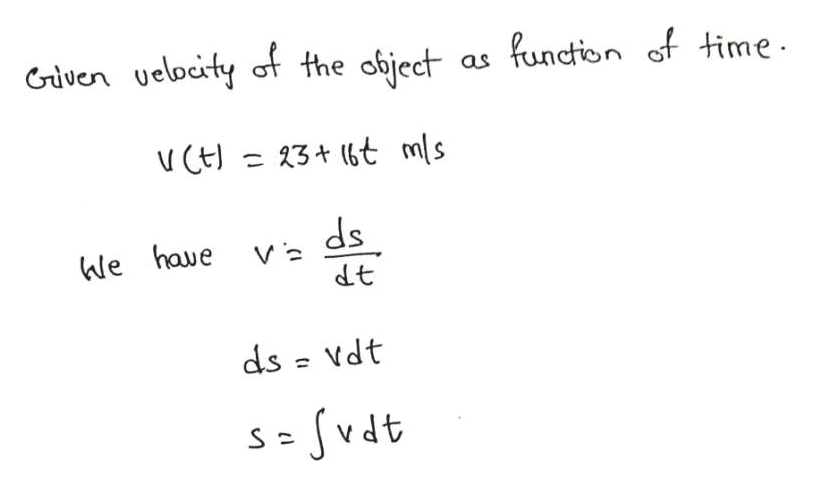# Given v(t) = 23 + 16 t, where v is in m/s and t is in s, use calculus to determine the total displacement from t1 = 1.5 s to t2 = 3.1 s.

Question

Given v(t) = 23 + 16 t, where v is in m/s and t is in s, use calculus to determine the total displacement from t1 = 1.5 s to t2 = 3.1 s.

check_circleExpert Solution
Step 1help_outlineImage TranscriptioncloseGiven ueloeity of the object function of time as 3+ (t mls V ds dt We haue ds = Vdt Svdt fullscreen

### Want to see the full answer?

See Solution

#### Want to see this answer and more?

Solutions are written by subject experts who are available 24/7. Questions are typically answered within 1 hour*

See Solution
*Response times may vary by subject and question
Tagged in

### Physics